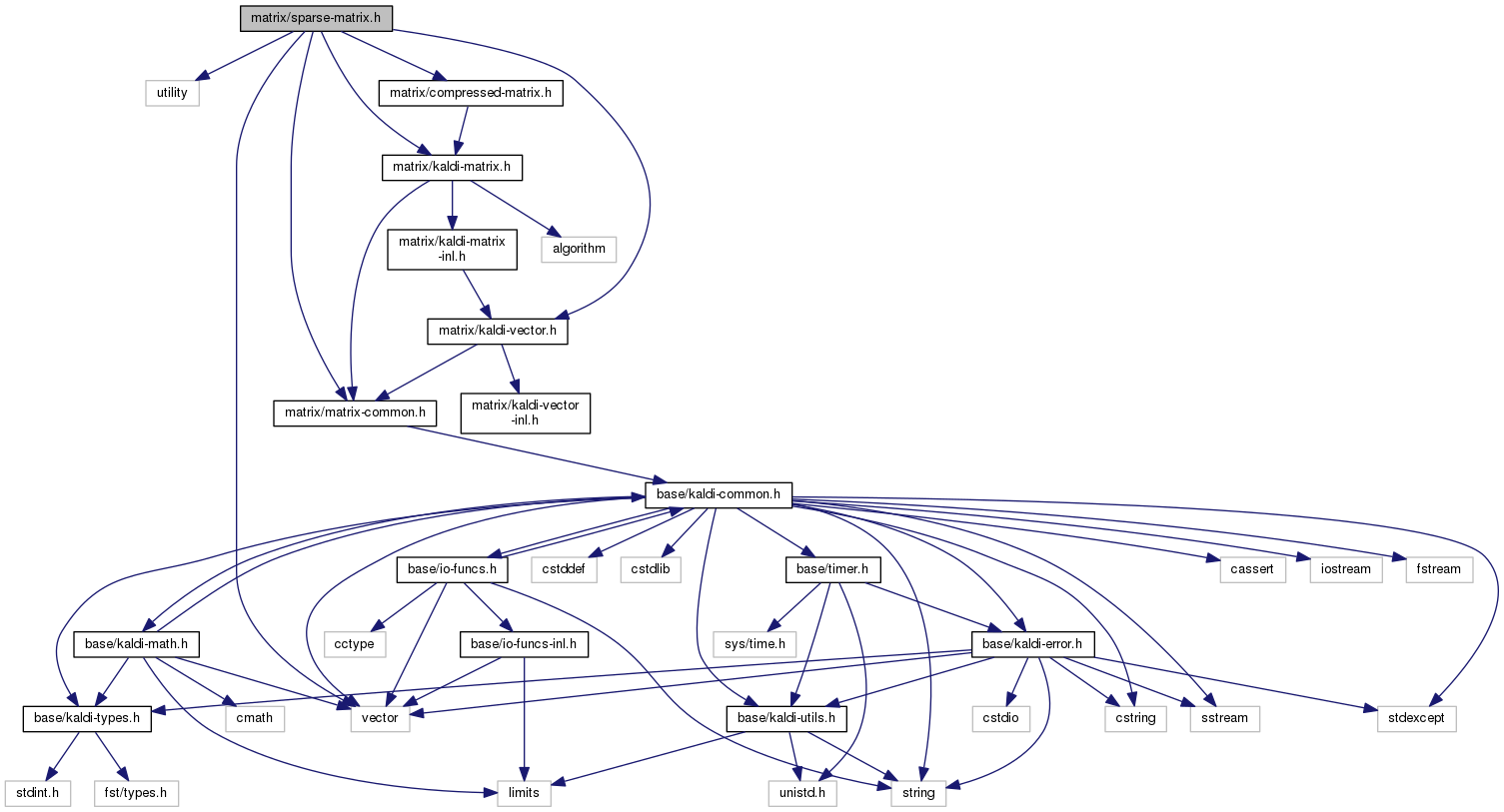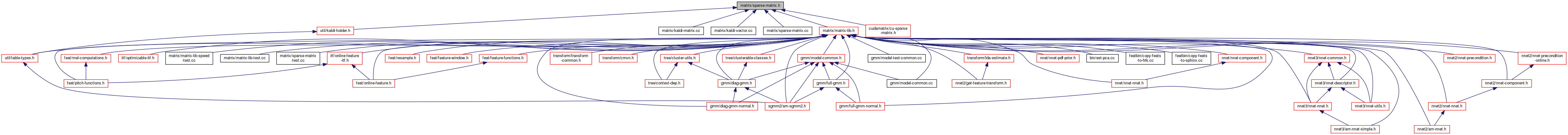sparse-matrix.h File Reference
`#include <utility>`
`#include <vector>`
`#include "matrix/matrix-common.h"`
`#include "matrix/kaldi-matrix.h"`
`#include "matrix/kaldi-vector.h"`
`#include "matrix/compressed-matrix.h"`
Include dependency graph for sparse-matrix.h:This graph shows which files directly or indirectly include this file:Go to the source code of this file.

## Classes

class  SparseVector< Real >

class  SparseMatrix< Real >

class  GeneralMatrix
This class is a wrapper that enables you to store a matrix in one of three forms: either as a Matrix<BaseFloat>, or a CompressedMatrix, or a SparseMatrix<BaseFloat>. More...

## Namespaces

kaldi
This code computes Goodness of Pronunciation (GOP) and extracts phone-level pronunciation feature for mispronunciations detection tasks, the reference:

## Enumerations

enum  GeneralMatrixType { kFullMatrix, kCompressedMatrix, kSparseMatrix }

## Functions

template<typename Real >
Real VecSvec (const VectorBase< Real > &vec, const SparseVector< Real > &svec)

template<typename Real >
Real TraceMatSmat (const MatrixBase< Real > &A, const SparseMatrix< Real > &B, MatrixTransposeType trans)

void AppendGeneralMatrixRows (const std::vector< const GeneralMatrix * > &src, GeneralMatrix *mat)
Appends all the matrix rows of a list of GeneralMatrixes, to get a single GeneralMatrix. More...

template<typename Real >
void FilterSparseMatrixRows (const SparseMatrix< Real > &in, const std::vector< bool > &keep_rows, SparseMatrix< Real > *out)
Outputs a SparseMatrix<Real> containing only the rows r of "in" such that keep_rows[r] == true. More...

template<typename Real >
void FilterMatrixRows (const Matrix< Real > &in, const std::vector< bool > &keep_rows, Matrix< Real > *out)
Outputs a Matrix<Real> containing only the rows r of "in" such that keep_keep_rows[r] == true. More...

void FilterCompressedMatrixRows (const CompressedMatrix &in, const std::vector< bool > &keep_rows, Matrix< BaseFloat > *out)
Outputs a Matrix<Real> containing only the rows r of "in" such that keep_rows[r] == true. More...

void FilterGeneralMatrixRows (const GeneralMatrix &in, const std::vector< bool > &keep_rows, GeneralMatrix *out)
Outputs a GeneralMatrix containing only the rows r of "in" such that keep_rows[r] == true. More...

void ExtractRowRangeWithPadding (const GeneralMatrix &in, int32 row_offset, int32 num_rows, GeneralMatrix *out)
This function extracts a row-range of a GeneralMatrix and writes as a GeneralMatrix containing the same type of underlying matrix. More...